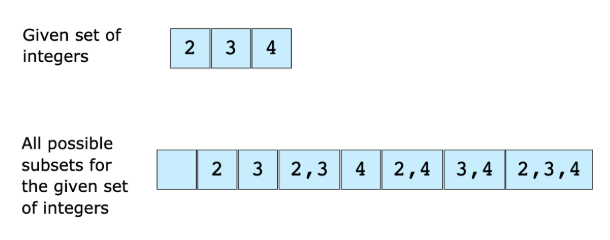# Find All Subsets

## Problem Statement

We are given a set of integers and we have to find all the possible subsets of this set of integers. The following example elaborates on this further.## Hint

• Guess the number of subsets for a set of size ‘n’
• Combinations

## Try it yourself

void get_all_subsets(vector<int>& v, vector<unordered_set<int>>& sets) {	    //TODO: Write - Your - Code}

## Solution

int get_bit(int num, int bit) {	int temp = (1 << bit);	temp = temp & num;	if (temp == 0) {		return 0;	}	return 1;}void get_all_subsets(vector<int>& v, vector<unordered_set<int>>& sets) {		int subsets_count = pow((double)2, (double)v.size());	for (int i = 0; i < subsets_count; ++i) {		unordered_set<int> set;		for (int j = 0; j < v.size(); ++j) {			if (get_bit(i, j)) {				set.insert(v[j]);			}		}		sets.push_back(set);	}}int main() {  int myints[] = {8,13,3,22, 17, 39, 87, 45, 36};	std::vector<int> v (myints, myints + sizeof(myints) / sizeof(int) );	vector<unordered_set<int>> subsets;	get_all_subsets(v, subsets);	cout << "****Total*****" << subsets.size() << endl;	for (int i = 0; i < subsets.size(); ++i) {		cout << "{";		for (unordered_set<int>::iterator it = subsets[i].begin(); it != subsets[i].end(); ++it) {			cout << *it << ",";		}		cout << "}" << endl;	}	cout << "****Total***** = " << subsets.size() << endl;	return 0;}

## Solution Explanation

### Runtime Complexity

Exponential, $O(2^{n}* n)$ - where ‘n’ is number of integers in the given set.

### Memory Complexity

Exponential, $O(2^{n}* n)$

### Solution Breakdown

There are several ways to solve this problem. We will discuss the one that is neat and easier to understand. We know that for a set of ‘n’ elements there are $2^{n}$ subsets. For example, a set with 3 elements will have 8 subsets. Here is the algorithm we will use:

n = size of given integer set
subsets_count = 2^n
for i = 0 to subsets_count
form a subset using the value of 'i' as following:
bits in number 'i' represent index of elements to choose from original set,
if a specific bit is 1 choose that number from original set and add it to current subset,
e.g. if i = 6 i.e 110 in binary means that 1st and 2nd elements in original array need to be picked.
add current subset to list of all subsets


Note that the ordering of bits for picking integers from the set does not matter; picking integers from left to right would produce the same output as picking integers from right to left.

Practice problems like this and many more by checking out our Grokking the Coding Interview: Patterns for Coding Questions course!

Learn in-demand tech skills in half the time